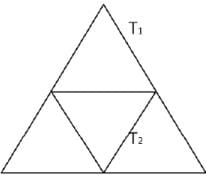Given an equilateral triangle T1 with side 24...

### Related TestGiven an equilateral triangle T1 with side 24 cm, a second triangle T2 is formed by joining the midpoints of the sides of T1. Then a third triangle T3 is formed by joining the midpoints of the sides of T2. If this process of forming triangles is continued, the sum of the areas, in sq cm, of infinitely many such triangles T1, T2, T3,... will be
• a)
188√3
• b)
248√3
• c)
164√3
• d)
192√3Nipuns InstituteWe can see that T2 is formed by using the mid points of T1. Hence, we can say that area of triangle of T2 will be (1/4)thof the area of triangle T1.Area of triangle T1 =√3 / 4 * (24)2 = 144√3 sq.cm
Area of triangle T2 = 144√3 / 4= 36√3 sq. cm
Sum of the area of all triangles = T1 + T2 + T3 + ...
=>T1 + T1 /4 + T1 /42 + ...
=> T1 / 1 – 0.25
=>4 / 3 * T1
=>4 / 3 * 144√3
=>192 √3
Hence, option D is the correct answer.View courses related to this question Explore CAT courses
 Explore CAT coursesView courses related to this question1 Crore+ students have signed up on EduRev. Have you?

• ### It normally takes 64 minutes to mow down an equilateral triangle shaped fie... more(Scan QR code)